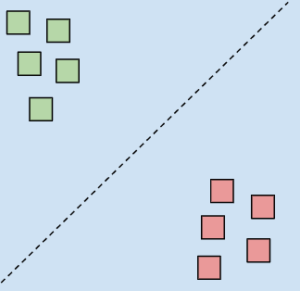Learnearn.uk » A Level Computer Science Home » Classification, Regression, Clustering & Reinforcement

# Classification, Regression, Clustering & Reinforcement

## Classification

### Classification

The aim of the classification is to split the data into two or more predefined groups. A common example is spam email filtering where emails are split into either spam or not spam.## Linear Regression

### Regression

The aim of the regression is to predict the value of a dependent variable based upon another explanatory variable. Linear Regression is used where there is a straight line correlation between variables, for example between poverty and teen birth rates.

## Non Linear Regression

Non Linear Regression

Non-linear regression is used where there is a correlation but it is not linear, for example between life expectancy and per capita income.

## Clustering

### Clustering

The objective of a clustering algorithm is to split the data into smaller groups or clusters based on certain features. The programmer might specify a target number of groups or let the algorithm decide.

## Reinforcement

### Reinforcement Learning

Reinforcement learning is a reward based system where an agent are not given specific instructions but are rewarded for how well they perform. Often this learning follows a Darwinian model where multiple agents attempt the task (each with different slightly randomly parameters) and those that perform the best form the base settings for their child (slightly mutated versions)

With each generation the agents improve in their performance.